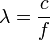# Frequency

Frequency is a property of an oscillation, vibration, or other regularly-repeating (cyclic) phenomenon. The frequency of such a phenomenon is the number of repetitions (cycles) in a unit of time. In the SI system of units, frequency is measured in Hertz (Hz), the number of repetitions in one second.

The frequency ( f ) of vibrations is inversely proportional to wavelength ( λ ), which is a length measurement:$\lambda = \frac{c}{f} \,\!$, where c is the speed of propagation of waves in the medium, or, for electromagnetic radiation, c = 299 792 458 m/s, the speed of light.

In electromagnetic radiation, the energy of the vibration is proportional to the frequency:$E=hf \,\!$, where h = 6.626 x 10-34 J•s (Planck's constant)Some content on this page may previously have appeared on Citizendium.